## 3D Coordinates (Q’s & Ans) || V.S.A.Q’S||

These solutions were designed by the ‘Basics in Maths‘ team. These notes to do help intermediate First-year Maths students.

Inter Maths – 1B two mark questions and solutions are very useful in IPE examinations.

### 3D Coordinates

1. Show that the points A (– 4, 9, 6), B (– 1, 6, 6), and C (0, 7, 10) form a right-angled isosceles triangle.

Sol:

Distance between two points P (x1, y1, z1) and Q (x2, y2, z2) is PQ =∴ The points A (– 4, 9, 6), B (– 1, 6, 6) and C (0, 7, 10) form a right-angled isosceles triangle.

2. Show that the locus of the point whose distance from Y – axis is thrice its distance from (1, 2, – 1) is 8x 2 + 9y2 + 8 z2 – 18x – 36y + 18z + 54 = 0

Sol:

Let P (x, y, z) be the locus of the point

A (0, y, 0) be any point on Y – axis

B = (1, 2, – 1)

Condition is PA = 3PB

PA2 = (3PB)2

PA2= 9 PB

⟹ x2 + z2 = 9[(x – 1)2 + (y – 2)2 + (z + 1)2]

x2 + z2 = 9[x2 – 2x + 1 + y2 – 4y + 4 + z2 + 2z + 1]

x2 + z2 = 9x2 – 18x + 9 + 9y2 – 36y + 36 +9 z2 + 18z + 9

∴ 8x 2 + 9y2 + 8 z2 – 18x – 36y + 18z + 54 = 0

3. A, B, and C are three points on OX, OY, and OZ respectively, at distances a, b, c (a≠0, b≠0, c≠0) from the origin ‘O’. Find the coordinate of the point which is equidistant from A, B, C, and O

Sol:

Let P (x, y, z) be the required point

O = (0, 0, 0)   A = (a, 0, 0), B = (0, b, 0), C = (0, 0, c)

Given, AP = BP = CP = OP

AP = OP

⟹   AP2 =OP2

(x – a )2 + y2 + z2 = x2 + y2 + z2

x2 + a2 – 2ax + y2 + z2 = x2 + y2 + z2

a2 – 2ax = 0

a (a – 2x) = 0

a – 2x = 0 (∵ a≠0)

a = 2x ⟹ a/2

Similarly, y = b/2 and z = c/2

∴ P = (a/2, b/2, c/2)

4. Show that the points A (3, – 2, 4), B (1, 1, 1) and C (– 1, 4, – 2) are collinear

Sol:

Given points are A (3, – 2, 4), B (1, 1, 1), and C (– 1, 4, – 2)5.Find x if the distance between (5, – 1, 7), (x, 5, 1) is 9 units.

Sol:

Let A = (5, – 1, 7), B = (x, 5, 1)

Given AB = 9

⟹ AB2 = 81

(5 – x)2 + (– 1 – 5)2 + (7 – 1)2 = 81

(5 – x)2 + 36 + 36 = 81

(5 – x)2 + 72 = 81

(5 – x)2 = 81 – 72 = 9

(5 – x) = ± 3

5 – x = 3 or 5 – x = – 3

5 – 3 = x or 5 + 3 = x

x = 2 or x = 8

6.If the point (1, 2, 3) is changed to point (2, 3, 1) through the translation of axes. Find a new origin.

Sol:

Given (x, y, z) = (1, 2, 3) and (X, Y, Z) = (2, 3, 1)

x = X + h, y = Y + k, z = Z + l

h = x – X, k = y – Y, l = z – Z

h = 1 – 2, k = 2 – 3, l = 3 – 1

h = – 1, k = – 1, l = 2

New origin is (– 1, – 1, 2)

7.By section formula, find the point which divides the line joining the points (2, – 3, 1) and (3, 4, – 5) in the ratio 1 : 3.

Sol:

If a point P divides the line segment joining the points (x1, y1, z1), (x2, y2, z2) in the ratio, thenLet P divides the line joining the points (2, – 3, 1) and (3, 4, – 5) in the ratio 1 : 38.Find the ratio in which the line joining two points (7, 0, – 1) and (– 2, 3, 5) is divided by the point (1,2,3).

Sol:

Let A = (7, 0, – 1), B = (– 2, 3, 5) and P = (1,2,3)

Suppose P divides AB in the ratio k : 19.Using section formula, verify whether the points A (2, –4, 3), B (–4, 5, 6), C (4, –7, 2) are collinear or not.

Sol:

Given Points are A (2, –4, 3), B (–4, 5, 6), C (4, –7, 2)

Let C divides AB in the ratio k : 12 – 4k = 4 (k + 1)

2 – 4k = 4k + 4

– 4k– 4k = 4 – 2

– 8k = 2

K = -1/4

C divides AB in the Ratio 1 : 4 externally

∴ A, B, C are collinear

10.Find the centroid of the triangle whose vertices are (5, 4, 6), (1, –1, 3) and (4, 3, 2)

Sol:

The centroid of the triangle whose vertices are (x1, y1, z1), (x2, y2, z2) and (x3, y3, z3) is11. Find the centroid of the tetrahedron whose vertices are (2, 3, –4), (–3, 3, –2), (–1, 4, 2) and (3, 5, 1)

Sol:

The centroid of the tetrahedron whose vertices are (x1, y1, z1), (x2, y2, z2) (x3, y3, z3), and (x4, y4, z4) is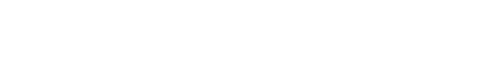the centroid of the tetrahedron whose vertices are (2, 3, –4), (–3, 3, –2), (–1, 4, 2) and (3, 5, 1) is12. Find the ratio in which the YZ-plane divides the line joining A(2, 4, 5) and B (3, 5, – 4)

Sol:

Let P be any point on the YZ-plane

P = (0, y, z)

Let P divides AB int eh ratio k:1YZ-plane divides AB in the ratio – 2:3

13. Find the fourth vertex of the parallelogram whose consecutive vertices are (2, 4, – 1), (3, 6, – 1) and (4, 5, 1).

Sol:

let A = (2, 4, – 1), B = (3, 6, – 1), C = (4, 5, 1) and D = (x, y, z)

ABCD is a parallelogram

Midpoint of AC = Midpoint of BD⟹ 3 + x = 6, 6 + y = 9, – 1 + z = 0

x = 3, y = 3, z = 1

∴ The fourth vertex D = (3, 3, 1)

14. A (5, 4, 6), B (1, –1, 3), and C (3, 3, 1) are three points. Find the coordinates of the point at which the bisector of ∠BAC meets the side BC.

Sol:

We know that the bisector of ∠BAC divides BC in the ratio AB: ACIf D is a point where the bisector of ∠BAC meets BC

⟹ D divides BC in the ratio 5:315. If M (α, β, γ) is the midpoint of the line joining the points (x1, y1, z1) and B, then find B

Sol:

Let B (x, y, z) be the required point

M is the midpoint of AB

⟹ (α, β, γ) =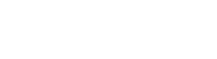⟹ 2 α = x + x1; 2 β = y + y1; 2 γ = z + z1

x =2 α – x1; x =2 β – y1; x =2 γ – z1

∴ B = (2 α – x1, 2 β – y1, 2 γ –)

16. If H, G, S, and I respectively denote the orthocenter, centroid, circumcenter, and incentre of a triangle formed by the points (1, 2, 3), (2, 3, 1) and (3, 1, 2), then find H, G, S, I.

Sol:

Let A = (1, 2, 3), B= (2, 3, 1), C = (3, 1, 2)⟹ ∆ ABC is an equilateral triangle

We know that, in an equilateral triangle orthocenter, centroid, circumcenter, and incentre are the same

Centroid G =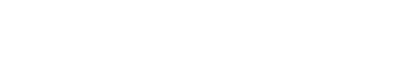= (2, 2, 2)

∴ H = (2, 2, 2), S = (2, 2, 2), I = (2, 2, 2)

17. Find the incentre of the triangle formed by the points (0, 0, 0), (3, 0, 0) and (0, 4, 0).

Sol:

Let A = (0, 0, 0), B = (3, 0, 0) and C = (0, 4, 0)∴ I = (1, 1,0)

18. Find the ratio in which point P (5, 4, – 6) divides the line segment joining the points A (3, 2, – 4) and B (9, 8, –10). Also, find the harmonic conjugate of P

Sol:

Harmonic Conjugate: If P divides AB in the ratio m: n, then the Harmonic Conjugate of P (i.e., Q) divides AB in the ratio –m : n.

Given points are A (3, 2, – 4), B (9, 8, –10), and P (5, 4, – 6)

P divides AB in the ratio is x2 – x : x – x2

= 3 – 5 : 5 – 9

= 1 : 2 (internally)

Let Q be the harmonic conjugate of P

⟹ Q divides AB in the ratio –1 : 2

Q =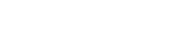= (–3, –4, –2)

Q (–3, –4, –2) is the hormonic conjugate of P (5, 4, – 6)

19. If (3, 2, – 1), (4, 1,1), and (6, 2, 5) are three vertices and (4, 2, 2) is the centroid of the tetrahedron, find the fourth Vertex.

Sol:

Given vertices of Tetrahedron are A (3, 2, – 1), B (4, 1,1), C (6, 2, 5) and centroid G = (4, 2, 2)

Let the fourth vertex is D = (x, y, z)

The centroid of the tetrahedron whose vertices are (x1, y1, z1), (x2, y2, z2) (x3, y3, z3), and (x4, y4, z4) is13 + x = 16, 5 + y = 8, 5 + z = 2

x = 3, y = 3, z = 3

∴ the fourth vertex D = (3, 3, 3)

20. Show that the points A(3, 2, –4), (5, 4, –6), and C(9, 8, –10) are collinear and find the ratio in which B divides AC.

Sol:

Given points are A(3, 2, –4), (5, 4, –6) and C(9, 8, –10)∴ points A (3, 2, –4), (5, 4, –6), and C (9, 8, –10) are collinear

B divides AB in the ratio is AB: BC =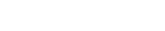##### Visit my Youtube Channel: Click on Below Logo